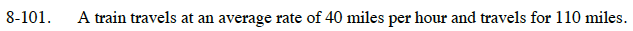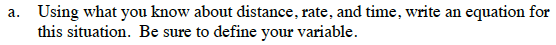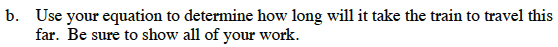Home > CC1 > Chapter 8 > Lesson 8.3.2 > Problem8-101

8-101.
1. A train travels at an average rate of 40 miles per hour and travels for 110 miles. Homework Help ✎

1. Using what you know about distance, rate, and time, write an equation for this situation. Be sure to define your variable.

2. Use your equation to determine how long will it take the train to travel this far. Be sure to show all of your work.If a train traveled at 30 miles per hour for 60 miles, it would take 2 hours based on the equation below.

$t= \frac{60}{30}$Based on the expression above, how long would it take to travel 110 miles at a rate of 40 miles per hour?

2.75 hours or about 2 hours, 45 minutes